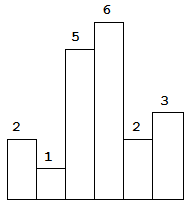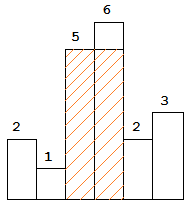# [LeetCode] 84. Largest Rectangle in Histogram 直方图中最大的矩形

Given n non-negative integers representing the histogram's bar height where the width of each bar is 1, find the area of largest rectangle in the histogram.Above is a histogram where width of each bar is 1, given height = [2,1,5,6,2,3].The largest rectangle is shown in the shaded area, which has area = 10 unit.

For example,
Given height = [2,1,5,6,2,3],
return 10.

// Pruning optimize
class Solution {
public:
int largestRectangleArea(vector<int> &height) {
int res = 0;
for (int i = 0; i < height.size(); ++i) {
if (i + 1 < height.size() && height[i] <= height[i + 1]) {
continue;
}
int minH = height[i];
for (int j = i; j >= 0; --j) {
minH = min(minH, height[j]);
int area = minH * (i - j + 1);
res = max(res, area);
}
}
return res;
}
};

class Solution {
public:
int largestRectangleArea(vector<int> &height) {
int res = 0;
stack<int> st;
height.push_back(0);
for (int i = 0; i < height.size(); ++i) {
if (st.empty() || height[st.top()] < height[i]) {
st.push(i);
} else {
int cur = st.top(); st.pop();
res = max(res, height[cur] * (st.empty() ? i : (i - st.top() - 1)));
--i;
}
}
return res;
}
};

class Solution {
public:
int largestRectangleArea(vector<int>& heights) {
int res = 0;
stack<int> st;
heights.push_back(0);
for (int i = 0; i < heights.size(); ++i) {
while (!st.empty() && heights[st.top()] >= heights[i]) {
int cur = st.top(); st.pop();
res = max(res, heights[cur] * (st.empty() ? i : (i - st.top() - 1)));
}
st.push(i);
}
return res;
}
};

Maximal Square

Maximal Rectangle

https://leetcode.com/problems/largest-rectangle-in-histogram/

https://leetcode.com/problems/largest-rectangle-in-histogram/discuss/28905/My-concise-C%2B%2B-solution-AC-90-ms

https://leetcode.com/problems/largest-rectangle-in-histogram/discuss/28902/5ms-O(n)-Java-solution-explained-(beats-96)

LeetCode All in One 题目讲解汇总(持续更新中...)

posted @ 2015-03-09 02:38  Grandyang  阅读(47929)  评论(15编辑  收藏  举报